Model-Building Problem Checklist

# Excel Applications for Accounting Principles

## Quiz 29 :Model-Building Problem ChecklistStudy FlashcardsLooking for Accounting Homework Help?## Quiz 29 :Model-Building Problem Checklist

Showing 1 - 20 of 32Exotic Adventures, a chain of stores servicing the ecotourism industry, has provided you with the following list of accounts and balances for the year ended June 30, 2012. All amounts shown are in thousands of dollars. You have been asked by the company's chief financial officer (CFO) to use a spreadsheet to prepare financial statements for Exotic Adventures, including an income statement, a statement of retained earnings, a classified balance sheet, and (if required by your instructor) a statement of cash flows. The CFO can't remember the accounts receivable balance. She asks you to figure it out when you prepare the balance sheet. The financial information presented here is accumulated in a file named FINANCIAL on the website for this book at cengagebrain.com. You are encouraged to use this file for preparing your statements and to use the information in this file as a Data Section for your answer. Place the statements in the space below the data. The financial statements should be shown in thousands of dollars just as in the Data Section. Exotic Adventures is a privately held company, so earnings per share information is not required.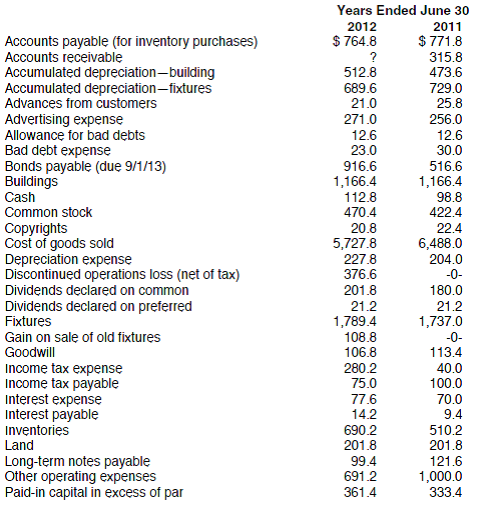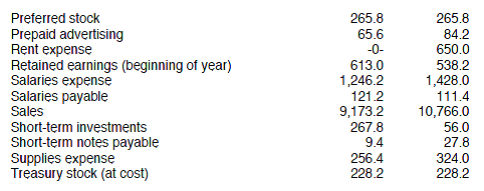Additional information required for the statement of cash flows: • Exotic Adventures sold a large amount of obsolete and worn-out fixtures for $128.8. The fixtures originally cost$248.0 and had been depreciated down to a net book value of $20.0. • Additional common stock, 24,000 shares, was issued for$76,000 in July. The par value of the stock is $2 per share. • Write off of goodwill and amortization of copyrights are included in other operating expenses. • No bonds were retired during the year. No additional notes payable were issued during the year. • Short-term investments of$220.0 were made during the year. Exotic Adventures also sold $8.2 short-term investments at no gain or loss. Review the Model-Building Problem Checklist on page 154 to ensure that your model is complete. When done, preview the printout to make sure that the worksheet will print neatly, and then print the worksheet. Check figure: Total assets,$3,385.2. To test your model, suppose the auditors made the following three adjustments to the June 30, 2012, balances: sales were reduced by $100,000 to 9,073.2; inventories were increased by$60,000 to 750.2; and accounts payable were increased by $160,000 to 924.8. Record these adjustments to your model. Does your balance sheet still balance Print the worksheet again. Check figure: Total assets,$3,445.2. CHART (optional) Using the test data worksheet, create a pie chart showing all major sources of funding for Exotic Adventures at year-end: current liabilities, long-term liabilities, and stockholders' equity. Print the chart when done.
Free
Essay

Financial statements
There are different types of financial statements prepared for accounting purpose like income statements, statement of retained earnings, balance sheet, statement of cash flow etc. These financial statements are used by owners, investors, banks, creditors, stock exchange, financial institutions etc.
Balance sheet shows all types of assets, all types of capitals, liabilities, loans balances etc. It shows financial position at a point of time which is closing date. Balance sheet is also called statement of financial position.
Prepare the financial statements before adjustments as shown below: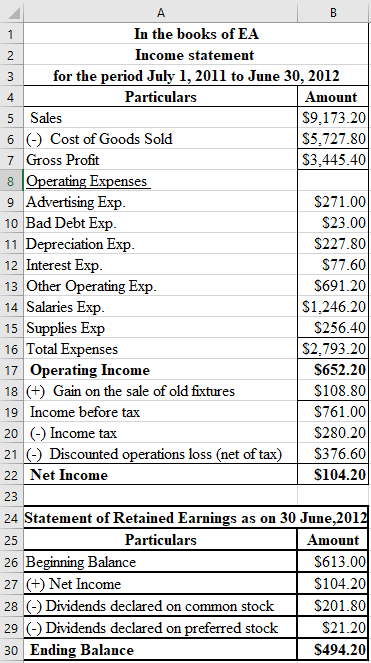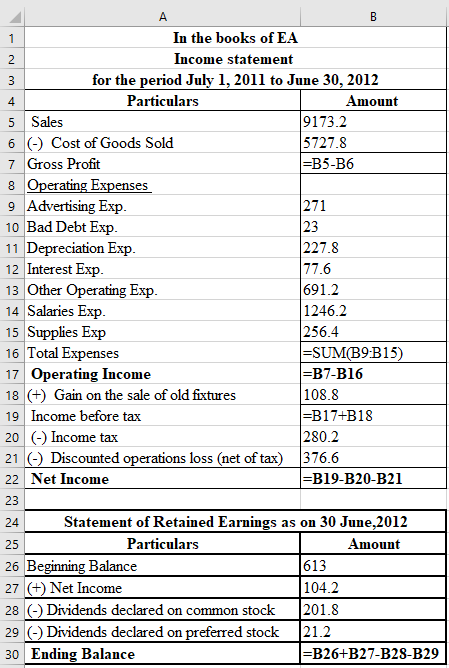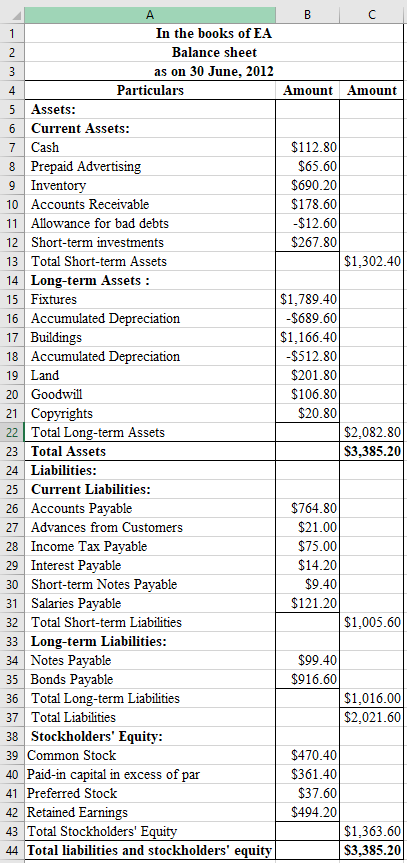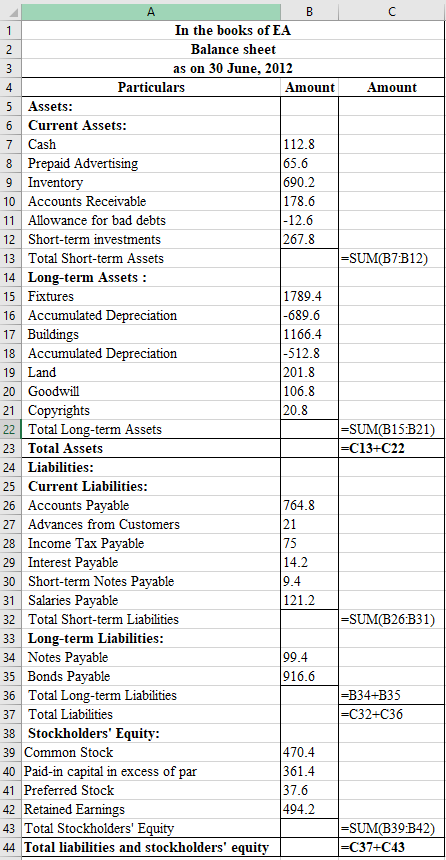Therefore, the net income and balance sheet totals before adjustments are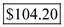and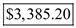respectively.
Prepare the financial statements after adjustments as shown below: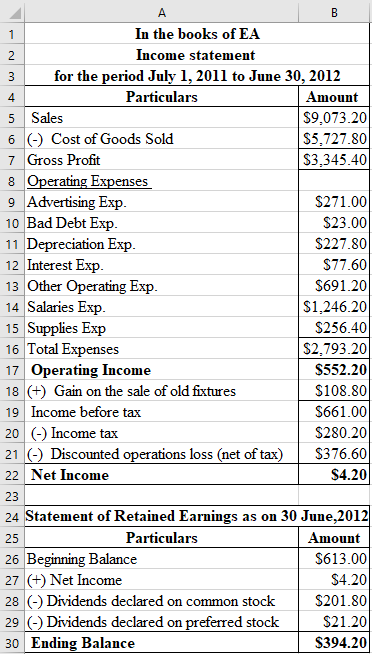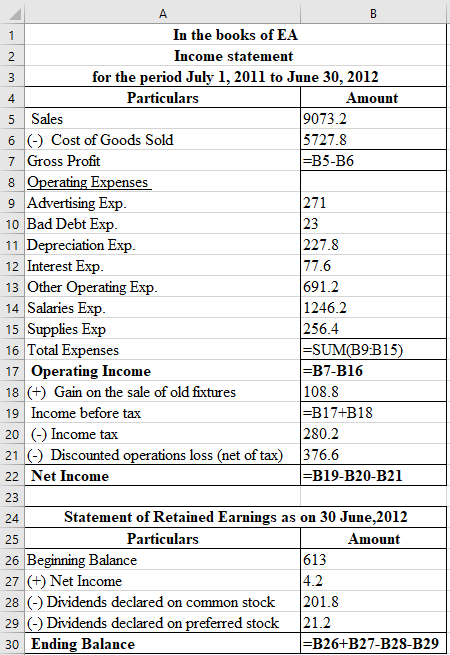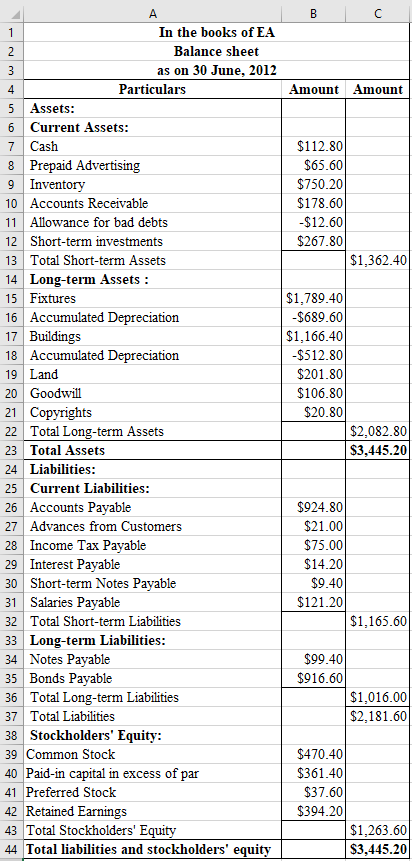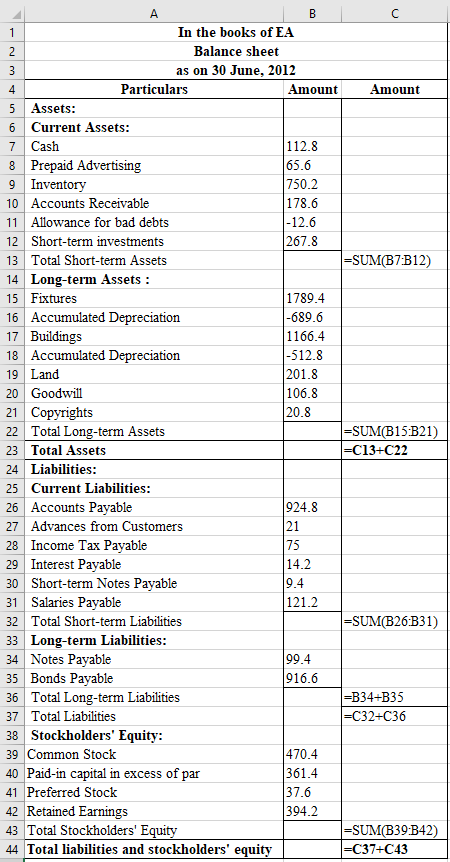Therefore, the net income and balance sheet totals before adjustments are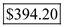and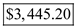respectively.
Prepare a pie chart based on the second set of data showing all major sources of funding for the company at year-end as shown below:
Total current liabilities are $1,165.6, long-term liabilities are$1,016, and stockholders' equity is equal to $1,263.6.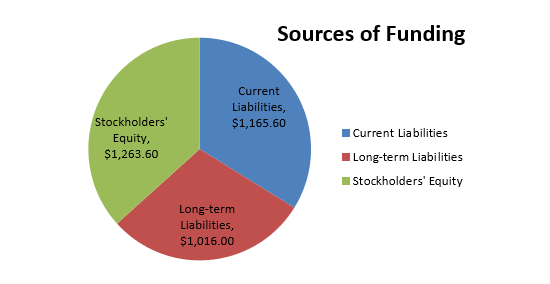Note: For a problem using the corporate form of business organization, download FTB from the website for this book found at cengagebrain.com. The general ledger of Jay Consulting shows the following balances at July 31: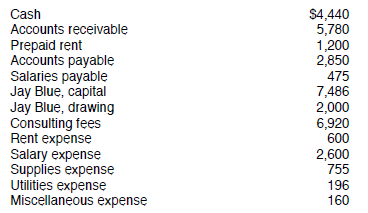Jay has asked you to develop a worksheet that will serve as a trial balance (file name PTB). Use the data provided as input for your model. Review the Model-Building Problem Checklist on page 154 to ensure that your worksheet is complete. Print the worksheet when done. Check figure: Total debits,$17,731. To test your model, use the following balances at August 31: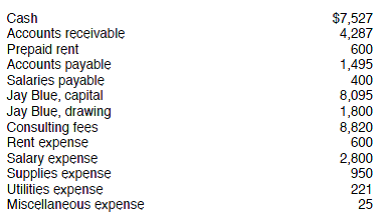Print the worksheet when done. Check figure: Total debits, $18,810. CHART (optional) Using the test data worksheet, prepare a pie chart showing the percentage of each asset to total assets. Print the chart when done. Free Essay Answer: Answer: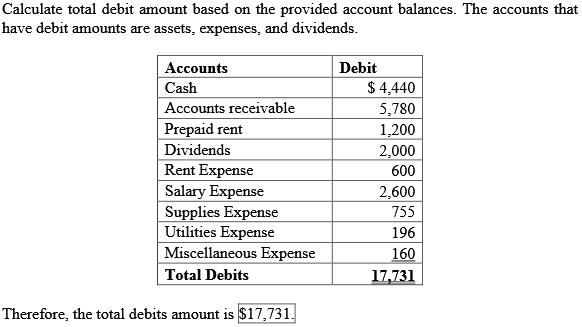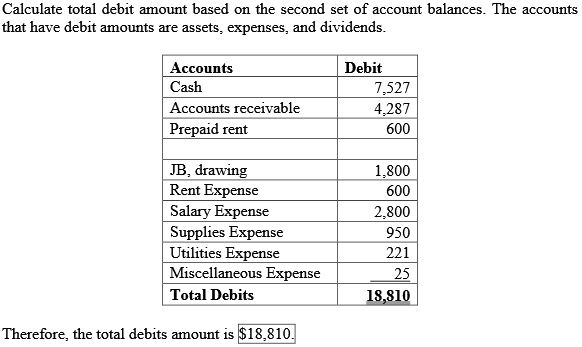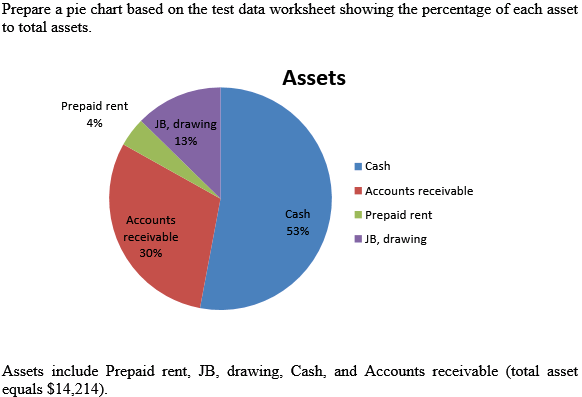The financial information presented here is accumulated in a file named ANALYSIS on the website for this book at cengagebrain.com. You are encouraged to use this file for preparing your analysis and to use the information in this file as a Data Section for your answer.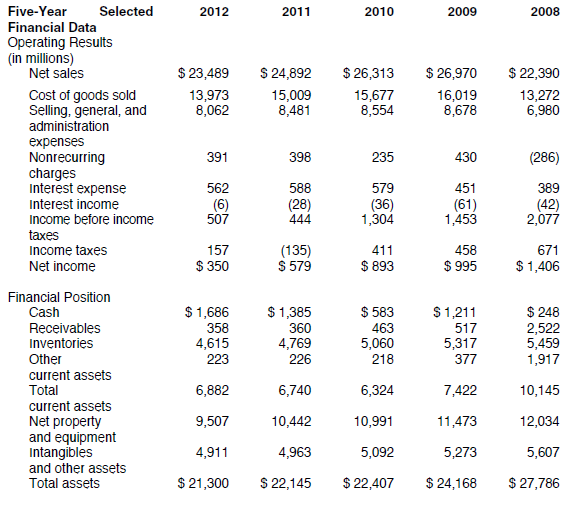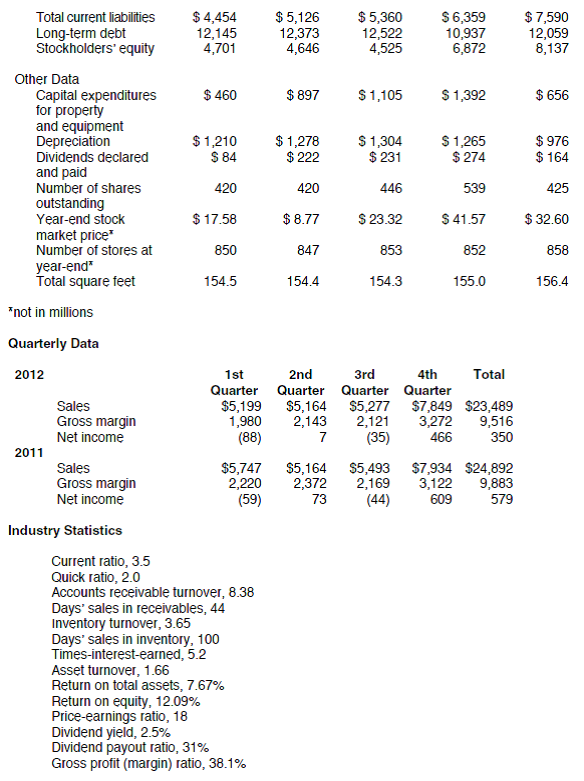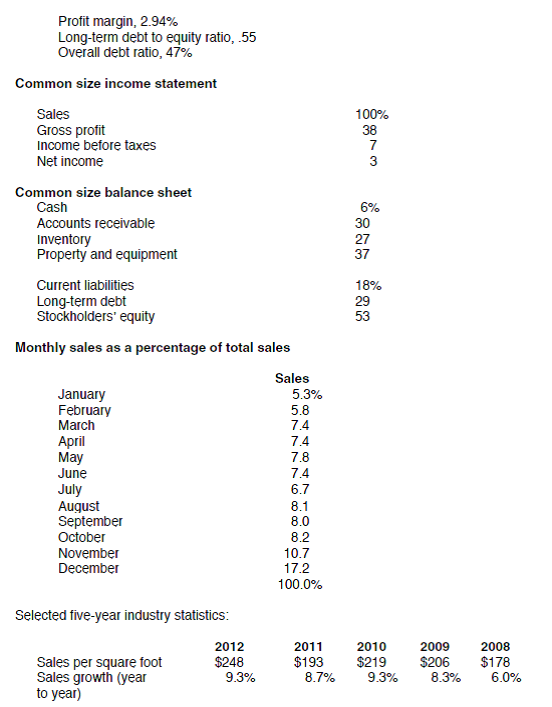Check off the following ratios that your instructor wants you to include in your report. You will want to group these ratios by type according to your textbook (profitability, liquidity, and so forth). Compute them for all five years. Some ratios require average balances rather than yearend figures. Since figures are not available for 2007, use year-end balances rather than average amounts for 2008 ratios. Lacy's has no preferred stock. Your instructor may also provide you with additional ratios or statistics to compute. Enter them at the bottom of each list. In your analysis, note Lacy's current position as well as important trends. Describe changes in profitability, liquidity, long-term solvency, and so forth. Evaluate these changes in light of the future. Compare ratios to industry standards. Standard Ratios and Statistics a. Working capital b. Current ratio c. Acid test (quick) ratio d. Accounts receivable turnover e. Number of days' sales in receivables f. Inventory turnover g. Number of days' sales in inventory h. Times interest earned i. Total asset turnover j. Rate of return on total assets k. Rate of return on stockholders' equity l. Earnings per share m. Dividends per share n. Price-earnings ratio o. Dividend yield p. Dividend payout ratio q. Gross profit (margin) ratio r. Profit margin (net income/sales) s. Long-term debt to equity ratio t. Overall debt ratio u. Book value per share v. w. x. Trends a. Sales b. Cost of goods sold c. Gross profit d. Selling, general, and administrative expenses e. Income before taxes f. Net income g. Other a. Sales per square foot b. Common size income statements (vertical analysis) c. Common size balance sheets (vertical analysis) d. Statement of cash flows for 2009-2012 e. Comparative income statements between years 2012 and 2011 (horizontal analysis-compute dollar change and percent change) f. Comparative balance sheets between years 2012 and 2011 (horizontal analysis-compute dollar change and percent change) g. Seasonal sales and income analysis (only two years available) Review the Model-Building Problem Checklist on page 154 to ensure that your model is complete and ready to be graded. When done, preview the printout to make sure that the worksheet will print neatly, and then print the worksheet. No check figures are provided. No test data are provided for this model. CHART (optional) Prepare a 3-D area chart comparing earnings per share and dividends per share over the fiveyear period. Print the chart when done. Free Essay Answer: Answer: Calculate the following ratios for all five years (from 2008 to 2012) using the given financial statements in the Excel file. Standard ratios and Statistics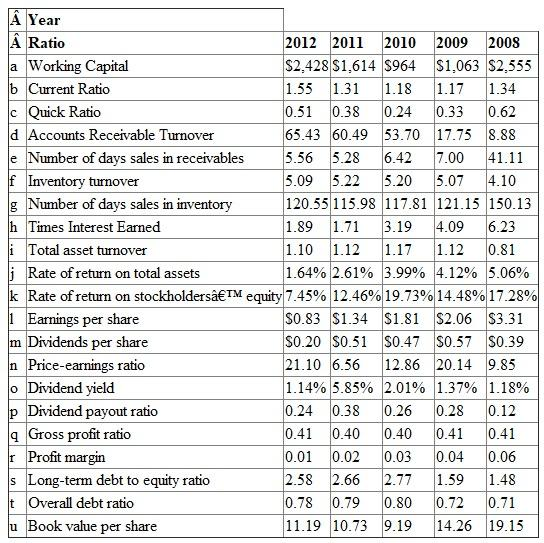Notes: 1. Working Capital is equal to current assets minus current liabilities. 2. Current ratio is equal to current assets divided by current liabilities. 3. Quick ratio is equal to current assets minus inventories divided by current liabilities. 4. Accounts receivable turnover is calculated by dividing net sales by the average account receivables. 5. The number of days' sales in receivables is calculated by dividing accounts receivables by net sales and multiplying by the number of days. In this case, 365 were used as the number of days. 6. Inventory turnover is calculated by dividing net sales by inventory. 7. The number of days' sales in inventory equals inventory divided by cost of goods sold and multiplied by the number of days. In this case, 365 was used as the number of days. 8. Times interest earned is calculated by dividing earnings before interest and tax by interest expense. 9. Total asset turnover equals net sales divided by total assets. 10. Rate of return on total assets equals net income divided by total assets. 11. Rate of return on stockholders' equity equals net income divided by stockholders' equity. 12. Earnings per share are calculated by dividing net income by the average of common shares outstanding. 13. Dividends per share are equal to dividends declared and paid divided by the average of common shares outstanding. 14. The price-earnings ratio equals market value per share divided by earnings per share. 15. The dividend yield equals dividends per share divided by price per share. 16. The dividend payout ratio equals dividends declared and paid divided by net income. 17. Gross profit ratio equals net sales minus cost of goods sold and divided by net sales. 18. Profit margin equals net income divided by net sales. 19. Long-term debt to equity ratio is calculated by dividing long-term debt by the stockholders' equity. 20. The overall debt ratio equals total debt divided by total assets. 21. Book value per share equals total stockholders' equity divided by the average of common shares outstanding. 22. The ratio analysis shows that the company's profitability has decreased over these five years as the return on asset decreased from 5.06% to 1.64% and the return on equity decreased from 17.28% to 7.45%. 23. Moreover, the company's solvency has worsened because the long-term debt to equity ratio has increased from 1.48 to 2.58 resulting in more debt compared to equity. On the other hand, the company's liquidity has improved as the current asset ratio rose from 1.34 to 1.55 meaning that the company has increased its current assets in respect to its current liabilities. Trends The following table shows how each of the following accounts has changed year-over-year. The year-over year change is calculated by subtracting the previous year's value from the current year's value and dividing by the previous' years value. Since data for 2007 is not provided, the year-over-year change for 2008 cannot be calculated. Note that Gross profit equals net sales minus cost of goods sold.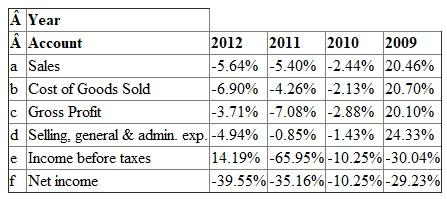Other (a) Sales per square feet are calculated by dividing net sales by total square feet.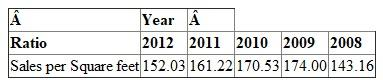(b) The common size income statements (vertical analysis) are calculated by converting all the amounts as percentage of net sales, this is done by dividing all the amounts by net sales. This has to be done for all five years using the each year's net sales.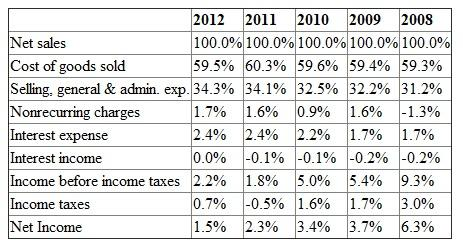(c) The common size balance sheets (vertical analysis) are calculated by converting all the amounts as percentage of total assets, this is done by dividing all the amounts by total assets. This has to be done for all five years using the each year's total assets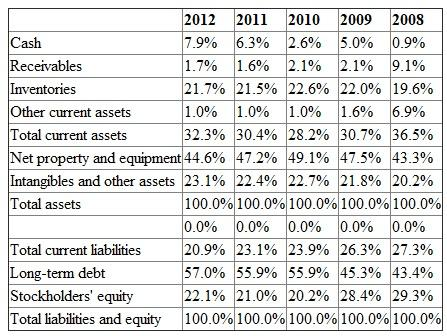. (d) Prepare a statement of cash flows for 2009-2012. Cash flow from operating activities is calculated by starting with net income of each year - Adding any non-cash expense, such as depreciation in the net income - Adding decreases in current assets and subtracting increases in current assets - Subtracting decreases in current liabilities and adding increases in current liabilities Note that changes in the cash account are not included in the calculation of cash flows from operating activities. Cash flows from financing activities include the dividends paid. Cash flows from operating activities include capital expenditures.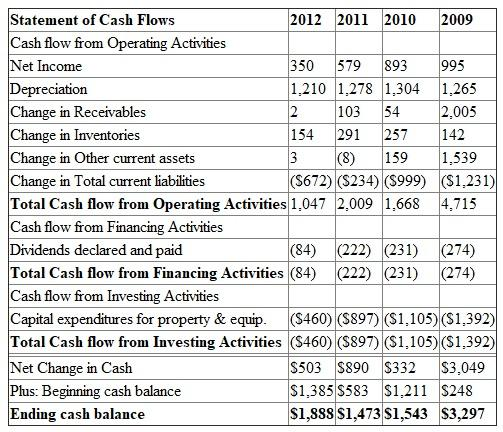(e) Compute comparative income statements between years 2012 and 2011 (horizontal analysis). The dollar change between years 2012 and 2011 is computed by subtracting the 2011 amounts from the 2012 amounts. The percentage change is calculated by subtracting the 2011 amounts from the 2012 amounts and dividing by the 2011 amounts.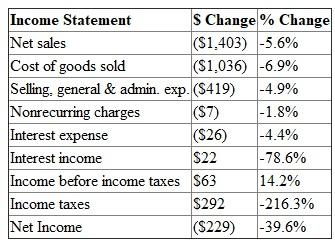(f) Compute comparative balance sheets between years 2012 and 2011 (horizontal analysis). The dollar change between years 2012 and 2011 is computed by subtracting the 2011 amounts from the 2012 amounts. The percentage change is calculated by subtracting the 2011 amounts from the 2012 amounts and dividing by the 2011 amounts.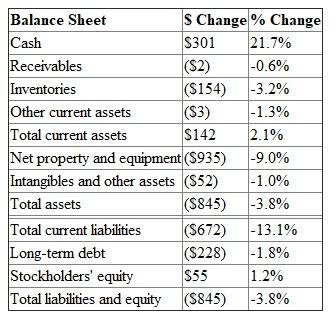(g) Compute the dollar change and percentage change of the quarterly results for years 2012 and 2011. The dollar change between years 2012 and 2011 is computed by subtracting the 2011 amounts from the 2012 amounts. The percentage change is calculated by subtracting the 2011 amounts from the 2012 amounts and dividing by the 2011 amounts.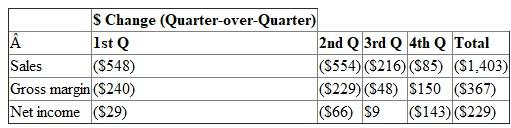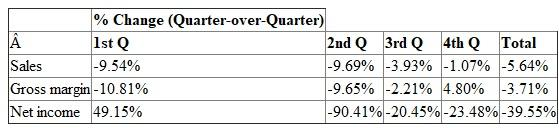Note: For a problem using the sole proprietorship form of business organization, download PFS from the website for this book found at cengagebrain.com. The ledger of Skitch Corp. showed the following balances after adjustment on June 30, 2012, the end of the current fiscal year: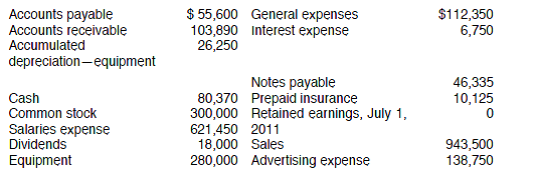The president of Skitch has asked you to develop a financial statement worksheet (file name FFS) that includes a single-step income statement, a statement of retained earnings, and a balance sheet. This worksheet will allow the financial statements to be prepared quickly by entering account balances in the appropriate cells on the worksheet. Use the information provided as input for your worksheet. Review the Model-Building Problem Checklist on page 154 to ensure that your worksheet is complete. Print the worksheet when done. Check figure: Total assets,$448,135. To test your model, use the following data for the year ended June 30, 2013: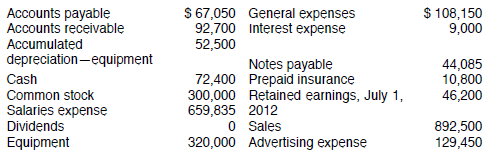Print the worksheet when done. Check figure: Total assets, $443,400. CHART (optional) Utilizing the test data worksheet, prepare a pie chart depicting the percentage of each expense to total expenses. Print the chart when done. Essay Answer:The balance sheet of Thorny Corp. as of December 31 is as follows: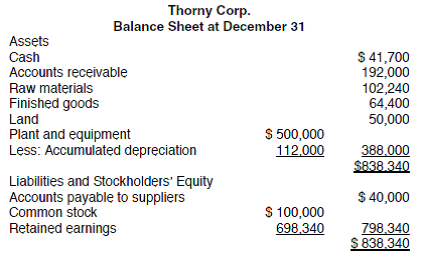The following information has been extracted from Thorny's records: 1. Thorny manufactures and sells Gizmos. The company has projected unit sales for its product for the next five months as follows: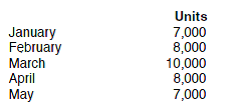All sales are made on account. Gizmos sell for$60 each. Forty percent of all sales are collected in the month of sale. The remaining 60% are collected in the following month. 2. Management desires to maintain the finished goods inventory for Gizmos at 20% of the following month's sales. Thorny's December 31 finished goods inventory consists of 1,400 Gizmos. 3. In order to produce one Gizmo, the following units of raw materials are used: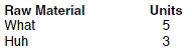The price of What has recently risen and is now $4 per unit. The price of Huh is$2.80 per unit. Management desires to maintain the ending raw materials inventory for both What and Huh at 25% of the following month's production needs. Thorny's December 31 raw material inventory consists of 18,000 units of What (@ $4.00 each) and 10,800 units of Huh (@$2.80 each). 4. Seventy percent of all purchases are paid in the month of purchase. The remaining 30% is paid in the subsequent month. 5. The company's product requires 30 minutes of direct labor time to complete. All labor costs are paid in the month incurred. Each hour of direct labor costs $24. 6. Factory overhead is applied at the rate of$12 per direct labor hour. Actual overhead costs are paid as they are incurred. Monthly differences between applied and actual overhead costs are expected to be negligible. 7. Selling and administrative expenses are $10,000 per month plus 10% of sales. They are paid in the month incurred. 8. Plant and equipment depreciates at the rate of$12,000 per year. This depreciation is incurred evenly throughout the year and is included in the factory overhead costs mentioned earlier. Design a worksheet (file name BIGBUD) for Thorny Corp. to prepare the following budgets for January, February, and March: sales budget, production budget, raw materials purchases budget, cash budget, projected unit cost to produce one Gizmo, budgeted income statement, and budgeted balance sheet. Use a column of the worksheet for each month and a final column for totals for the quarter. Round budget calculations (except unit costs) to the nearest dollar. The sales budget and raw materials purchases budgets should be expressed both in units and in dollars. Thorny uses FIFO cost flow assumption for valuing inventories. The acid test for developing this model: When you are done, are your balance sheets in balance Review the Model-Building Problem Checklist on page 154 to ensure that your worksheet is complete and ready to be graded. When done, preview the printout to make sure that the worksheet will print neatly, and then print the worksheet. Check figure: Total assets at the end of March, $1,036,858. To test your model, increase the price of Gizmo to$70, change March sales units to 11,000, increase the direct labor time to complete a Gizmo to 36 minutes, and increase the variable selling and administrative expense rate to 15%. Now hold your breath. Does your worksheet calculate throughout and do your balance sheets balance Print the worksheet again. Check figure: Total assets at the end of March, $1,104,010. CHART (optional) Using the test data, create a 3-D area chart that compares monthly cash flow (change in the cash account) with monthly net income. Print the chart when done. Not Answered There is no answer for this questionThe terms of sales on account offered by Cubberly's Farm Supplies are 2/10, n/30. During the first week of June, the following transactions involved receiving cash: June 1 Received$450.80 from Dylan Seikel in payment of a May 26 invoice of $460, less discount. 3 Received a loan of$8,000 from First National Bank. 4 Received $245.00 from Alicia Geddes in payment of a May 14 invoice. 5 Received$500.78 from Bingham Corp. in payment of a May 28 invoice of $511, less discount. 7 Cash sales for the week totaled$15,600. Cubberly's Farm Supplies has asked you to create a computerized cash receipts journal (file name CRJ). The journal should include one column each for the Date, Account Name, Other Accounts Cr., Sales Cr., Accounts Receivable Cr., Sales Discount Dr., and Cash Dr. The appropriate columns should be totaled. Use the data for the first week in June as input for the worksheet. Review the Model-Building Problem Checklist on page 154 to ensure that your worksheet is complete. Print the worksheet when done. Check figure: Total debits, $24,816.00. To test your model, use the following information for the second week of June: June 8 Received$426.30 from Alicia Geddes in payment of a June 1 invoice of $435, less discount. 9 Received$896.70 from King World in payment of a May 28 invoice of $915, less discount. 12 Received$712.75 from McDonald Farms in payment of a June 1 invoice. 13 Received $350 for old store equipment that had no book value. 14 Cash sales for the week totaled$14,222. Print the worksheet when done. Check figure: Total debits, $16,634.75. CHART (optional) Using your test model, create a 3-D column chart showing the amount of cash Cubberly's Farm Supplies collected from each credit customer the second week of June. Print the chart when done. Essay Answer:DuBridge, Inc., has the following portfolio of trading securities at December 31, 2013. All shares were purchased during 2013.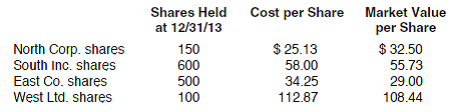Develop a worksheet (file name STI) that will automatically compute the total cost of stock in the portfolio and the total market value. Further, the worksheet should state "Unrealized Gain" or "Unrealized Loss" for each stock, as appropriate. To accomplish this, use the =IF function discussed in Appendix A of Excel Quick. The worksheet should also disclose the value of the portfolio to be shown on the December 31, 2013, balance sheet. Use the data above as input for your model. Review the Model-Building Problem Checklist on page 154 to ensure that your worksheet is complete. Print the worksheet when done. Check figure: Balance sheet value at 12/31/13,$63,657.00. Extra assignment: Prepare the adjusting journal entry required at 12/31/13. Use the following 12/31/14 market value to test your model: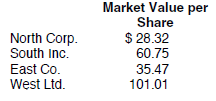Print the worksheet when done. Check figure: Balance sheet value at 12/31/14, $68,534.00. Extra assignment: Prepare the adjusting journal entry required at 12/31/14. CHART (optional) Using the test model, prepare a pie chart showing the percentage that the market value for each security is of the total portfolio. Print the chart when done. Essay Answer:Beginning inventory, purchases, and sales data for Part Number CKW2 are presented below. A perpetual inventory account is maintained for this part using the weighted-average costing method. Develop a worksheet (file name PERPTL) to be used as a perpetual inventory record for this part. Your worksheet should include columns for date, quantity purchased, total cost of units purchased, quantity sold, total cost of units sold, quantity balance, total cost balance, and weighted-average unit cost.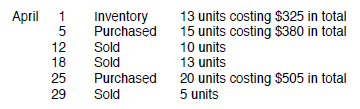Review the Model-Building Problem Checklist on page 154 to ensure that your worksheet is complete. Print the worksheet when done. Check figure: Ending inventory balance,$504.71. To test your model, record the following transactions for May: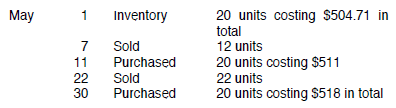Print the worksheet when done. Check figure: Ending inventory balance, $670.76. CHART (optional) Create a line chart on the test data template showing the trend in weighted-average cost per unit after each purchase over the two-month period. Essay Answer:Quality Reclining Chairs has inventory at June 30 as follows: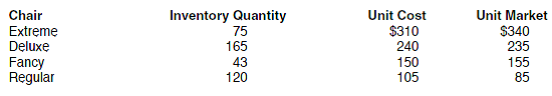You have been asked to develop a worksheet that will determine the value of the inventory on an item-by-item basis at the lower of cost or market value (file name LCOM). You should find it helpful to use the =MIN function discussed in Appendix A of Excel Quick. Use the provided data as input for your worksheet. Review the Model-Building Problem Checklist on page 154 to ensure that your worksheet is complete. Print the worksheet when done. Check figure: Lower of cost or market inventory value,$78,675. To test your model, use the following information about Quality Reclining Chairs inventory at September 30: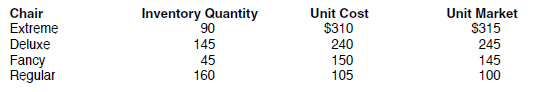Print the worksheet when done. Check figure: Lower of cost or market inventory value, $85,225. CHART (optional) From the test data worksheet, design a 3-D column chart demonstrating the relationship between total cost and total market value for each commodity. Print the chart when done. Essay Answer:Peach Management owns several apartment complexes throughout the city of Atlanta. It just acquired a new piece of property that has been appraised at$775,000. The assets and their values are listed as follows.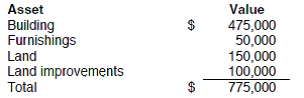Peach Management purchased the property in a foreclosure for $600,000. Create a worksheet (file name BASKET) that will allocate the purchase price among the assets acquired. Review the Model-Building Problem Checklist on page 154 to ensure that your worksheet is complete. Print the worksheet when done. Check figure: Allocated cost of the building,$367,742. Use the following purchase price to test your model: $700,000. Print the worksheet when done. Check figure: Allocated cost of the building at the purchase price of$700,000, $429,032. CHART (optional) Using the data template, prepare a pie chart that shows each asset's percentage of the total appraised value. Print the chart when done. Essay Answer:Rolly Corporation is considering different methods of financing a$1,200,000 expansion of plant facilities. At present, there are 140,000 shares of $20 par value common stock outstanding. One financing plan under consideration calls for issuing an additional 40,000 shares of$20 par value common stock at $25 per share and$200,000 of 10-year, 8% bonds at face value. The president has asked you to develop a worksheet that will calculate expected earnings per share (file name NEWMONEY) if this financing plan is adopted. Your worksheet should include a Data Section. Net income before bond interest is expected to be $1,000,000. Assume an income tax rate of 40%. Review the Model-Building Problem Checklist on page 154 to ensure that your worksheet is complete. Print the worksheet when done. Check figure: Earnings per share,$3.28. To test your model, calculate expected earnings per share if $1,200,000 of 10-year, 8% bonds are issued at face value and no new shares of stock are issued. Check figure: Earnings per share,$3.87. CHART (optional) Using the test data worksheet, create a line chart to show the trend in earnings per share as the portion of bond financing changes from $0 to$1,200,000. Use $400,000 increments. Assume that the total to be financed is$1,200,000 and that common stock (at $25 per share) will be issued to cover any amount not financed by bonds. Print the chart. Essay Answer:MacDuff and MacBeth recently formed a partnership called Inverness Ltd. MacDuff invested$30,000 cash, and MacBeth invested $30,000 of plant assets and$15,000 cash. The partners are trying to find an equitable way of splitting the income to take into account that MacBeth invested more capital, but MacDuff will spend twice as much time as MacBeth in running the business. They have asked you to develop a worksheet (file name PSHIP) to allow them to see the effect of: • various interest rates on invested capital • various salary allowances for time spent • various levels of partnership net income The easiest way to achieve this flexibility is to use a Data Section. Use the following information as input for your model: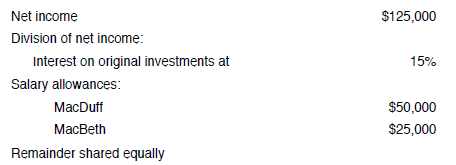Review the Model-Building Problem Checklist on page 154 to ensure that your worksheet is complete. Print the worksheet when done. Check figure: MacDuff's share of income, $73,875. To test your model, use the following profit-sharing scenario: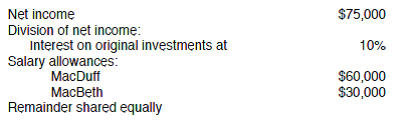Print the worksheet when done. Check figure: MacDuff's share of income,$51,750. CHART (optional) Using the test data provided, prepare a 3-D stacked column chart showing the division of net income (salary, interest, and remainder) to each partner. Print the chart when done.
EssayThe following data were selected from the records of Magnus Incorporated for the month ended February 28: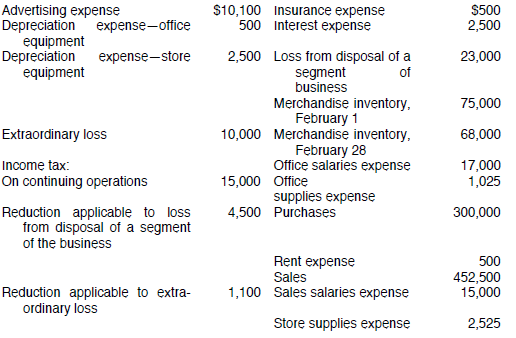This firm's accounting system generates these totals on a monthly basis. You have been asked to develop a worksheet (file name IS) that will allow a monthly income statement to be prepared by entering monthly figures in the appropriate cells. The income statement should include a section for earnings per share. There were 20,000 shares of common stock (no preferred) outstanding throughout the month. Use the provided data as input for your worksheet. Review the Model-Building Problem Checklist on page 154 to ensure that your worksheet is complete. Print the worksheet when done. Check figure: February net income, $50,950. To test your model, use the following data for the month ended March 31: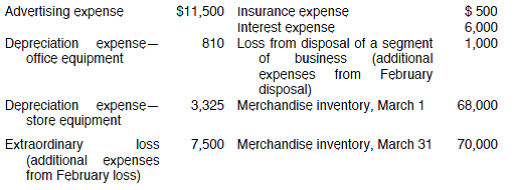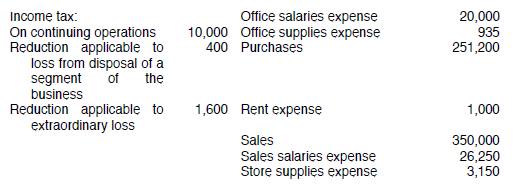There was no change in the number of shares of common stock outstanding. Print the worksheet when done. Check figure: March net income,$10,920. CHART (optional) From the test data worksheet, develop a pie chart that presents the percentage distribution of selling expenses. Print the chart when done.
EssayA prospective investor has hired you to develop a worksheet that will compute the book value per share (file name BVALUE) on each class of stock of various companies. Your worksheet should include a Data Section. One of the companies the investor is currently interested in is Hawk Enterprises, which has the following capital structure: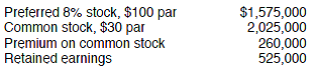Preferred stock has a prior claim to assets on liquidation to the extent of 105% of par. Review the Model-Building Problem Checklist on page 154 to ensure that your worksheet is complete. Print the worksheet when done. Check figure: Book value per share, Hawk Enterprises: Common stock, $40.46. To test your model, use the following data for Crow Corporation:Preferred stock has a prior claim to assets on liquidation to the extent of 110% of par. Print the worksheet when done. Check figure: Book value per share, Crow Corporation: Common stock,$1.41. CHART (optional) Using the test worksheet, prepare a pie chart that shows how much of the total equity is allocated to preferred stock and how much is allocated to common stock. Print the chart when done.
EssayDifferences in accounting methods between those applied to its accounts and those used in determining taxable income yielded the following amounts for the first three years of Fancy Schmansy, Inc.: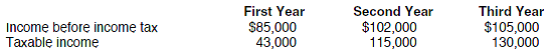The income tax rate for each of the three years was 40% of taxable income, and taxes were paid promptly each year. The president of Fancy Schmansy has asked you to develop a worksheet (file name DEFERTAX) that will show income tax deducted on the income statement, income tax payments for the year, year's addition to (deduction from) deferred income tax payable, and the year-end balance of deferred income tax payable. Use the information provided as input for the Data Section of your worksheet. Review the Model-Building Problem Checklist on page 154 to ensure that your worksheet is complete. Print the worksheet when done. Check figure: Fancy Schmansy, year-end balance of deferred tax payable, third year, $1,600. The president of Superduper Enterprises is impressed with your model and wishes to test it using the following data from her company (assume a 35% tax rate):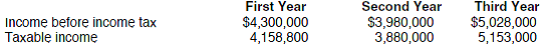Print the worksheet when done. Check figure: Superduper Enterprises year-end balance of deferred tax payable, third year,$40,670. CHART (optional) Using the Superduper Enterprises data, create a 3-D column chart showing the annual addition to or reduction in deferred taxes for all three years. Print the chart when done.
EssayOn July 1, Hanover Company issued $2,000,000 of 10-year, 6% bonds at an effective interest rate of 5%. This netted the company$2,154,435. Interest on the bonds is payable annually on July 1. The president of Hanover has asked you to develop an amortization schedule worksheet (file name AMORT) that will use the effective interest method to calculate annual interest expense, premium (or discount) amortization, unamortized premium (or discount), and bond carrying amount. Your worksheet should include a Data Section. Review the Model-Building Problem Checklist on page 154 to ensure that your worksheet is complete. Print the worksheet when done. Check figure: Amortization of bond premium, tenth year, $19,048. To test your model, calculate the annual interest expense, discount amortization, unamortized discount, and bond carrying amount of$700,000 of 10-year, 7.5% bonds at an effective interest rate of 8%. The issuance of these bonds netted the company $676,515. Interest on the bonds is payable annually. Print the worksheet when done. Check figure: Amortization of bond discount, tenth year,$3,241. CHART (optional) With information from the test data, create a 3-D area chart that shows annual interest paid and interest expense over the 10-year life of the bond. Print the chart when done.
EssayShanna Company reported a net income of $105,000 for the current year. Depreciation recorded for the year totaled$38,000. Current asset and liability accounts had the following balances at the beginning and end of the year: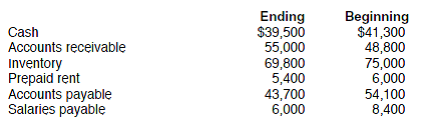The president of Shanna Company has asked you to develop a worksheet that will compute cash flow from operating activities (file name CASHFL). Use the information provided as input for the Data Section of your worksheet. Review the Model-Building Problem Checklist on page 154 to ensure that your worksheet is complete and ready to be graded. Print the worksheet when done. Check figure: Cash flow from operating activities, $129,800. To test your model, use the following information for the next year, when net income was$60,000 and depreciation totaled $30,000.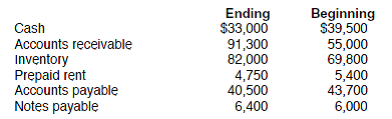Print the worksheet when done. Check figure: Cash flow from operating activities,$39,350. CHART (optional) Using the test model data, design a column chart that will show the components of cash flow from operating activities (net income, change in accounts receivable, etc.). Print the chart when done.
EssayOdie manages several rental properties for real estate investors. Each month, he reviews the cash flow statements from each property to look for trouble spots. Information for one of the properties, Eastwick Apartments, is as follows for June of the current year: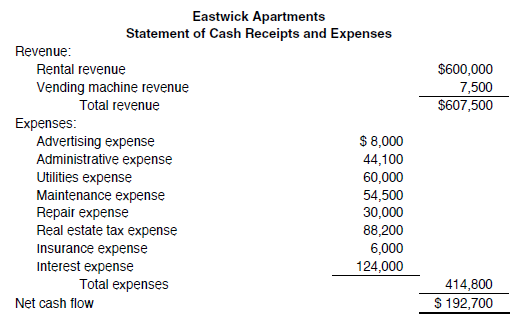Odie has asked you to develop a worksheet that will automatically prepare a vertical analysis of the cash flow data (file name VERT). All percentages should be based on total revenue. Use the data provided as input for your model. Review the Model-Building Problem Checklist on page 154 to ensure that your worksheet is complete. Print the worksheet when done. Check figure: Interest expense is 20% of total revenue. To test your model, use the following information for Westwick Apartments for June.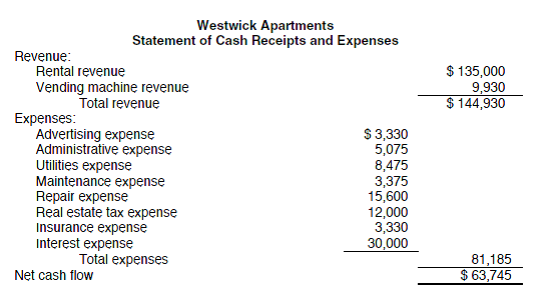Print the worksheet when done. Check figure: Interest expense is 21% of total revenue. CHART (optional) Using the test data worksheet, prepare a pie chart of the expenses. Print the chart when done.
EssayHelena Industries uses FIFO process costing in accounting for its production activities. Materials are added at the beginning of the process and conversion costs are incurred uniformly throughout the process. May's production records indicate the following information: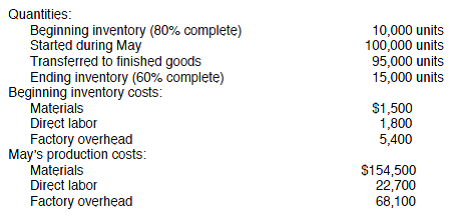Design a cost of production report worksheet (file name FIFOPRC) for Helena that can be used by the company at the end of each month. Your worksheet should include a Data Section. Use the May data as input for your report. Review the Model-Building Problem Checklist on page 154 to ensure that your worksheet is complete and ready to be graded. Print the worksheet when done. Check figure: Total equivalent unit cost in May, $2.49. Using the following data for June, test your model: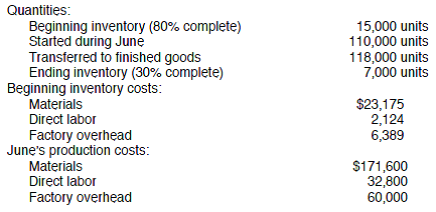Print the worksheet when done. Check figure: Total equivalent unit cost in June,$2.40. CHART (optional) Prepare a stacked bar chart that shows the proportionate amount of materials, labor, and overhead each month that make up the cost of each completed unit. Print the chart when done.Zylo Industries uses weighted-average process costing in accounting for its production activities. Materials are added at the beginning of the process and conversion costs are incurred uniformly throughout the process. August's production records indicate the following information: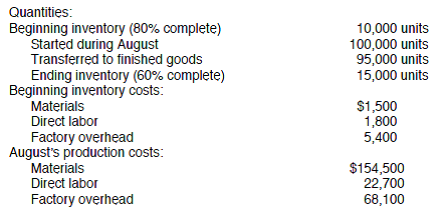Design a cost of production report template (file name WAPRC) for Zylo that can be used by the company at the end of each month. Your worksheet should include a Data Section. Use the August data as input for your report. Review the Model-Building Problem Checklist on page 154 to ensure that your worksheet is complete and ready to be graded. Print the worksheet when done. Check figure: Total equivalent unit cost in August, $2.36. Using the following data for September, test your model: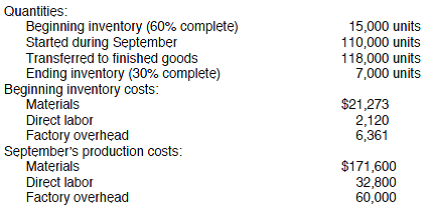Print the worksheet when done. Check figure: Total equivalent unit cost in September,$2.39. CHART (optional) Using the test model data, prepare a pie chart that shows the proportionate amount of materials, labor, and overhead that make up the cost of each completed unit. Print the chart when done.